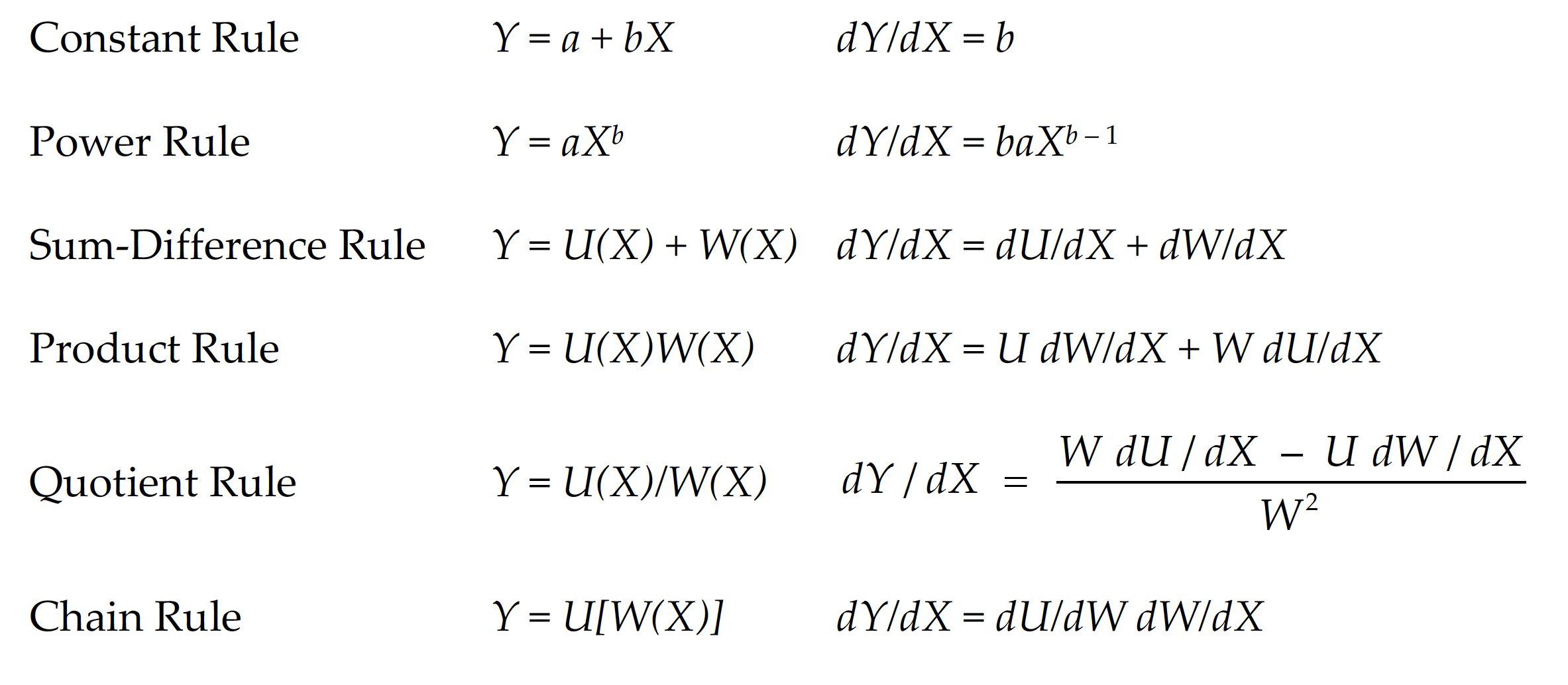# Rules for calculating (math) derivatives

Here’s a particularly useful list of rules for calculating (math) derivatives: